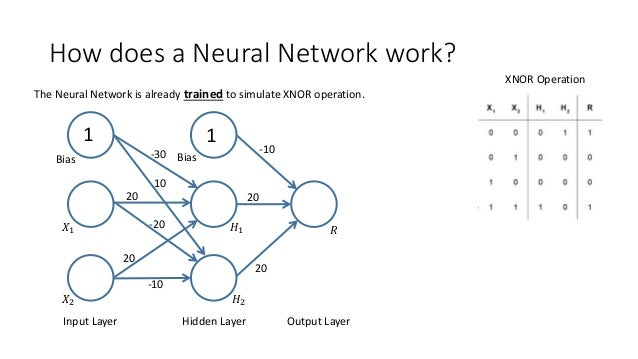Successfully reported this slideshow.Upcoming SlideShare
×

# How does a Neural Network work?

491 views

Published on

How does a Neural Network work is part of JavaLand talk. Find more info on https://goo.gl/rrLd1N

Published in: Data & Analytics
• Full Name
Comment goes here.

Are you sure you want to Yes No• Be the first to comment

### How does a Neural Network work?

1. 1. How does a Neural Network work? 1 1 Bias 𝑋1 𝑋2 𝐻1 𝐻2 Bias-30 20 20 10 -20 -10 -10 20 20 𝑅 Input Layer Hidden Layer Output Layer XNOR Operation The Neural Network is already trained to simulate XNOR operation.
2. 2. How does a Neural Network work? 1 1 Bias 𝑋1 𝑋2 𝐻1 𝐻2 Bias-30 20 20 -10 20 20 𝑅 Input Layer Hidden Layer Output Layer W𝐡𝐚𝐭 𝐢𝐬 𝐭𝐡𝐞 𝐯𝐚𝐥𝐮𝐞 𝐟𝐨𝐫 𝐇 𝟏, 𝐰𝐡𝐞𝐧 𝐗 𝟏 = 𝟎 𝐚𝐧𝐝 𝐗 𝟐 = 𝟎? 𝐻1= -30 + 20𝑋1 + 20𝑋2 = -30 -> 0 0 0 0 XNOR Operation
3. 3. How does a Neural Network work? 1 1 Bias 𝑋1 𝑋2 𝐻1 𝐻2 Bias 10 -20 -10 -10 20 20 𝑅 Input Layer Hidden Layer Output Layer W𝐡𝐚𝐭 𝐢𝐬 𝐭𝐡𝐞 𝐯𝐚𝐥𝐮𝐞 𝐟𝐨𝐫 𝐇 𝟐, 𝐰𝐡𝐞𝐧 𝐗 𝟏 = 𝟎 𝐚𝐧𝐝 𝐗 𝟐 = 𝟎? 𝐻2= 10 - 20𝑋1 - 10𝑋2 = 10 -> 1 0 0 0 1 XNOR Operation
4. 4. How does a Neural Network work? 1 1 Bias 𝑋1 𝑋2 𝐻1 𝐻2 Bias-30 20 20 10 -20 -10 -10 20 20 𝑅 Input Layer Hidden Layer Output Layer W𝐡𝐚𝐭 𝐢𝐬 𝐭𝐡𝐞 𝐯𝐚𝐥𝐮𝐞 𝐟𝐨𝐫 𝐑 , 𝐰𝐡𝐞𝐧 𝐗 𝟏 = 𝟎 𝐚𝐧𝐝 𝐗 𝟐 = 𝟎? 0 0 0 1 𝑅 = -10 + 20𝐻1 + 20𝐻2 = 10 -> 1 1 XNOR Operation
5. 5. How does a Neural Network work? 1 1 Bias 𝑋1 𝑋2 𝐻1 𝐻2 Bias-30 20 20 -10 20 20 𝑅 Input Layer Hidden Layer Output Layer W𝐡𝐚𝐭 𝐢𝐬 𝐭𝐡𝐞 𝐯𝐚𝐥𝐮𝐞 𝐟𝐨𝐫 𝐇 𝟏, 𝐰𝐡𝐞𝐧 𝐗 𝟏 = 𝟎 𝐚𝐧𝐝 𝐗 𝟐 = 𝟏? 𝐻1= -30 + 20𝑋1 + 20𝑋2 = -10 -> 0 0 0 1 XNOR Operation
6. 6. How does a Neural Network work? 1 1 Bias 𝑋1 𝑋2 𝐻1 𝐻2 Bias 10 -20 -10 -10 20 20 𝑅 Input Layer Hidden Layer Output Layer W𝐡𝐚𝐭 𝐢𝐬 𝐭𝐡𝐞 𝐯𝐚𝐥𝐮𝐞 𝐟𝐨𝐫 𝐇 𝟐, 𝐰𝐡𝐞𝐧 𝐗 𝟏 = 𝟎 𝐚𝐧𝐝 𝐗 𝟐 = 𝟏? 𝐻2= 10 - 20𝑋1 - 10𝑋2 = 0 -> 0 0 0 1 0 XNOR Operation
7. 7. How does a Neural Network work? 1 1 Bias 𝑋1 𝑋2 𝐻1 𝐻2 Bias-30 20 20 10 -20 -10 -10 20 20 𝑅 Input Layer Hidden Layer Output Layer W𝐡𝐚𝐭 𝐢𝐬 𝐭𝐡𝐞 𝐯𝐚𝐥𝐮𝐞 𝐟𝐨𝐫 𝐑 , 𝐰𝐡𝐞𝐧 𝐗 𝟏 = 𝟎 𝐚𝐧𝐝 𝐗 𝟐 = 𝟏? 0 0 1 0 𝑅 = -10 + 20𝐻1 + 20𝐻2 = -10 -> 0 0 XNOR Operation
8. 8. How does a Neural Network (NN) work? 1 1 Bias 𝑋1 𝑋2 𝐻1 𝐻2 Bias-30 20 20 -10 20 20 𝑅 Input Layer Hidden Layer Output Layer W𝐡𝐚𝐭 𝐢𝐬 𝐭𝐡𝐞 𝐯𝐚𝐥𝐮𝐞 𝐟𝐨𝐫 𝐇 𝟏, 𝐰𝐡𝐞𝐧 𝐗 𝟏 = 𝟏 𝐚𝐧𝐝 𝐗 𝟐 = 𝟎? 𝐻1= -30 + 20𝑋1 + 20𝑋2 = -10 -> 0 1 0 0 XNOR Operation
9. 9. How does a Neural Network work? 1 1 Bias 𝑋1 𝑋2 𝐻1 𝐻2 Bias 10 -20 -10 -10 20 20 𝑅 Input Layer Hidden Layer Output Layer W𝐡𝐚𝐭 𝐢𝐬 𝐭𝐡𝐞 𝐯𝐚𝐥𝐮𝐞 𝐟𝐨𝐫 𝐇 𝟐, 𝐰𝐡𝐞𝐧 𝐗 𝟏 = 𝟏 𝐚𝐧𝐝 𝐗 𝟐 = 𝟎? 𝐻2= 10 - 20𝑋1 - 10𝑋2 = -10 -> 0 1 0 0 0 XNOR Operation
10. 10. How does a Neural Network work? 1 1 Bias 𝑋1 𝑋2 𝐻1 𝐻2 Bias-30 20 20 10 -20 -10 -10 20 20 𝑅 Input Layer Hidden Layer Output Layer W𝐡𝐚𝐭 𝐢𝐬 𝐭𝐡𝐞 𝐯𝐚𝐥𝐮𝐞 𝐟𝐨𝐫 𝐑 , 𝐰𝐡𝐞𝐧 𝐗 𝟏 = 𝟏 𝐚𝐧𝐝 𝐗 𝟐 = 𝟎? 1 0 0 0 𝑅 = -10 + 20𝐻1 + 20𝐻2 = -10 -> 0 0 XNOR Operation
11. 11. How does a Neural Network work? 1 1 Bias 𝑋1 𝑋2 𝐻1 𝐻2 Bias-30 20 20 -10 20 20 𝑅 Input Layer Hidden Layer Output Layer W𝐡𝐚𝐭 𝐢𝐬 𝐭𝐡𝐞 𝐯𝐚𝐥𝐮𝐞 𝐟𝐨𝐫 𝐇 𝟏, 𝐰𝐡𝐞𝐧 𝐗 𝟏 = 𝟏 𝐚𝐧𝐝 𝐗 𝟐 = 𝟏? 𝐻1= -30 + 20𝑋1 + 20𝑋2 = 10 -> 1 1 1 1 XNOR Operation
12. 12. How does a Neural Network work? 1 1 Bias 𝑋1 𝑋2 𝐻1 𝐻2 Bias 10 -20 -10 -10 20 20 𝑅 Input Layer Hidden Layer Output Layer W𝐡𝐚𝐭 𝐢𝐬 𝐭𝐡𝐞 𝐯𝐚𝐥𝐮𝐞 𝐟𝐨𝐫 𝐇 𝟐, 𝐰𝐡𝐞𝐧 𝐗 𝟏 = 𝟏 𝐚𝐧𝐝 𝐗 𝟐 =1? 𝐻2= 10 - 20𝑋1 - 10𝑋2 = -20 -> 0 1 1 1 0 XNOR Operation
13. 13. How does a Neural Network work? 1 1 Bias 𝑋1 𝑋2 𝐻1 𝐻2 Bias-30 20 20 10 -20 -10 -10 20 20 𝑅 Input Layer Hidden Layer Output Layer W𝐡𝐚𝐭 𝐢𝐬 𝐭𝐡𝐞 𝐯𝐚𝐥𝐮𝐞 𝐟𝐨𝐫 𝐑 , 𝐰𝐡𝐞𝐧 𝐗 𝟏 = 𝟏 𝐚𝐧𝐝 𝐗 𝟐 = 𝟏? 1 1 1 0 𝑅 = -10 + 20𝐻1 + 20𝐻2 = 10 -> 1 0 XNOR Operation
14. 14. How does a Neural Network work? 1 1 Bias 𝑋1 𝑋2 𝐻1 𝐻2 Bias-30 20 20 10 -20 -10 -10 20 20 𝑅 Input Layer Hidden Layer Output Layer How do you train a Neural Network? 1 1 1 0 1 1) The edges are initialized with random weights. 2) A 𝑋1, 𝑋2 pair is given as un input. The network produces an erronous 𝑅 𝐹. Based on the Error 𝑒 = |𝑅 − 𝑅 𝐹| the edges are updated from right to the left in a way so that a renewed input of 𝑋1, 𝑋2 would produce 𝑅 𝐹 that is closer to 𝑅, thus the error 𝑒 is smaller. 3) Step 2 is executed for all pairs 𝑋1, 𝑋2 until the error 𝑒 is small enough for the network to be usable.